# 如何用不到 2KB的JavaScript代码写一个3D赛车游戏？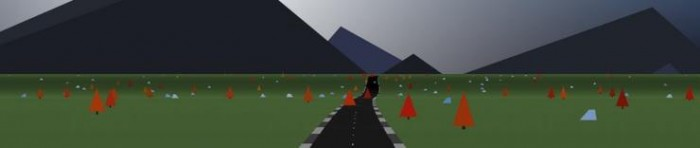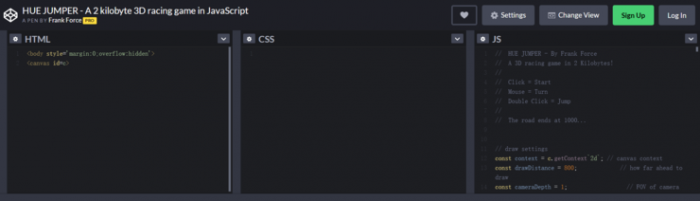HTML

<body 9em impact';         // set font size

context.fillStyle = LSHA(99,0,0,.5); // set font color

context.fillText(text, posX, 129);   // fill text

context.lineWidth = 3;               // line width

context.strokeText(text, posX, 129); // outline text

}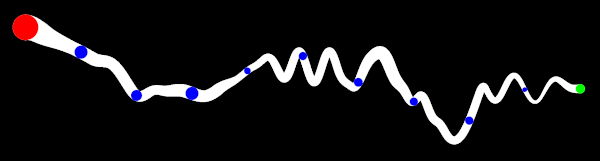atan2 函数可以用来计算道路俯仰角，据此来设计物理运动和光线。

roadGenLengthMax =                     // end of section

roadGenTaper =                         // length of taper

roadGenFreqX =                         // X wave frequency

roadGenFreqY =                         // Y wave frequency

roadGenScaleX =                        // X wave amplitude

roadGenScaleY = 0;                     // Y wave amplitude

startRandSeed = randSeed = Date.now(); // set random seed

for( i = 0; i < roadEnd*2; ++i )          // build road past end

{

{

// calculate difficulty percent

d = Math.min(1, i/maxDifficultySegment);

roadGenFreqX = R(Lerp(d,.01,.02));               // X curves

roadGenFreqY = R(Lerp(d,.01,.03));               // Y bumps

roadGenScaleY = R(Lerp(d,1e3,2e3));              // Y scale

// apply taper and move back

roadGenTaper = R(99, 1e3)|0;                 // random taper

roadGenLength = 0;                           // reset length

i -= roadGenTaper;                           // subtract taper

}

// apply taper from last section and lerp values

}

// reset everything

velocity = new Vec3

( pitchSpring =  pitchSpringSpeed =  pitchRoad = hueShift = 0 );

position = new Vec3(0, height);      // set player start pos

nextCheckPoint = checkPointDistance; // init next checkpoint

time = maxTime;                      // set the start time

Update=()=>

{

s = position.z / segmentLength | 0; // current road segment

p = position.z / segmentLength % 1; // percent along segment

// get lerped values between last and current road segment

// update player velocity

velocity.y += gravity;

velocity.x *= lateralDamp;

velocity.z = Math.max(0, time?forwardDamp*velocity.z:0);

// limit player x position (how far off road)

position.x = Clamp(position.x, -maxPlayerX, maxPlayerX);

// check if on ground

{

position.y = roadY; // match y to ground plane

airFrame = 0;       // reset air frames

// get the dot product of the ground normal and the velocity

// bounce velocity against ground normal

// apply player brake and accel

velocity.z +=

mouseDown? playerBrake :

Lerp(velocity.z/maxSpeed, mousePressed*playerAccel, 0);

{

velocity.z *= offRoadDamp;                    // slow down

pitchSpring += Math.sin(position.z/99)**4/99; // rumble

}

}

// update player turning and apply centrifugal force

turn = Lerp(velocity.z/maxSpeed, mouseX * turnControl, 0);

velocity.x +=

velocity.z * turn -

velocity.z ** 2 * centrifugal * roadX;

// update jump

if (airFrame++<6 && time

&& mouseDown && mouseUpFrames && mouseUpFrames<9)

{

velocity.y += jumpAccel; // apply jump velocity

airFrame = 9;            // prevent jumping again

}

mouseUpFrames = mouseDown? 0 : mouseUpFrames+1;

// pitch down with vertical velocity when in air

pitchSpringSpeed += Lerp(airPercent, 0, velocity.y/4e4);

// update player pitch spring

pitchSpringSpeed += (velocity.z - lastVelocity.z)/2e3;

pitchSpringSpeed -= pitchSpring * springConstant;

pitchSpringSpeed *= pitchSpringDamp;

pitchSpring += pitchSpringSpeed;

// was checkpoint crossed?

if (position.z > nextCheckPoint)

{

time += checkPointTime;               // add more time

nextCheckPoint += checkPointDistance; // set next checkpoint

hueShift += 36;                       // shift hue

}

// clear the screen and set size

c.width = window.innerWidth, c.height = window.innerHeight;

// calculate projection scale, flip y// get horizon, offset, and light amount

horizon = c.height/2 - Math.tan(playerPitch)*projectScale.y;

// create linear gradient for sky

// draw sky as full screen poly

DrawPoly(c.width/2,0,c.width/2,c.width/2,c.height,c.width/2,g);

// draw sun and moon (0=sun, 1=moon)

for( i = 2 ; i--; )

{

x = c.width*(.5+Lerp(

4, -4)-backgroundOffset),

y = horizon - c.width/5,

c.width/25,

x, y, i?c.width/23:c.width);

// draw full screen poly

DrawPoly(c.width/2,0,c.width/2,c.width/2,c.height,c.width/2,g);

}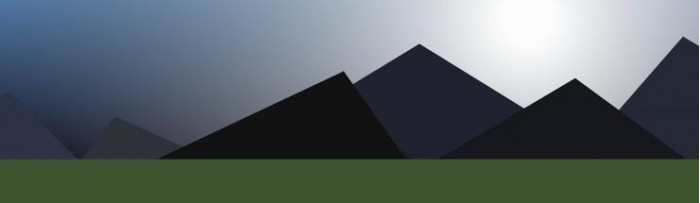// set random seed for mountains

randSeed = startRandSeed;

// draw mountains

for( i = mountainCount; i--; )

{

DrawPoly(

x = c.width*(.5+Lerp(angle/PI/2+.5,4,-4)-backgroundOffset),

y = horizon,

w = R(.2,.8)**2*c.width/2,

x + w*R(-.5,.5),

y - R(.5,.8)*w, 0,

LSHA(R(15,25)+i/3-light*9, i/2+R(19), R(220,230)));

}

// draw horizon

DrawPoly(

c.width/2, horizon, c.width/2, c.width/2, c.height, c.width/2,

LSHA(25, 30, 95));

for( x = w = i = 0; i < drawDistance+1; )

{

.Add(position.Multiply(-1));    // get local camera space

// tilt camera pitch and invert z

z = 1/(p.z*Math.cos(playerPitch) - p.y*Math.sin(playerPitch));

p.y = p.y*Math.cos(playerPitch) - p.z*Math.sin(playerPitch);

p.z = z;

// project road segment to canvas space

p.Multiply(new Vec3(z, z, 1))         // projection

.Multiply(projectScale)               // scale

.Add(new Vec3(c.width/2,c.height/2)); // center on canvas

}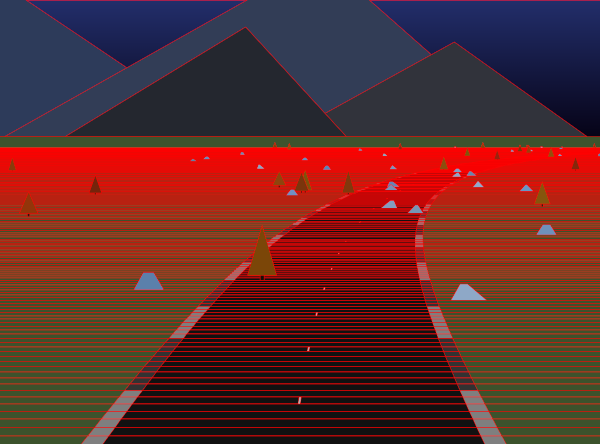let segment2 = road[s+drawDistance]; // store the last segment

for( i = drawDistance; i--; )        // iterate in reverse

{

p1 = segment1.p;

p2 = segment2.p;

// random seed and lighting

randSeed = startRandSeed + s + i;

light = Math.sin(segment1.a) * Math.cos(heading) * 99;

// check near and far clip

if (p1.z < 1e5 && p1.z > 0)

{

if (i % (Lerp(i/drawDistance,1,9)|0) == 0)

{

// ground

DrawPoly(c.width/2, p1.y, c.width/2,

c.width/2, p2.y, c.width/2,

LSHA(25 + light, 30, 95));

// curb if wide enough

if (segment1.w > 400)

DrawPoly(p1.x, p1.y, p1.z*(segment1.w+curbWidth),

p2.x, p2.y, p2.z*(segment2.w+curbWidth),

LSHA(((s+i)%19<9? 50: 20) + light));

DrawPoly(p1.x, p1.y, p1.z*segment1.w,

p2.x, p2.y, p2.z*segment2.w,

LSHA(((s+i)*segmentLength%checkPointDistance < 300 ?

70 : 7) + light));

// dashed lines if wide and close enough

if ((segment1.w > 300) && (s+i)%9==0 && i < drawDistance/3)

DrawPoly(p1.x, p1.y, p1.z*dashLineWidth,

p2.x, p2.y, p2.z*dashLineWidth,

LSHA(70 + light));

// save this segment

segment2 = segment1;

}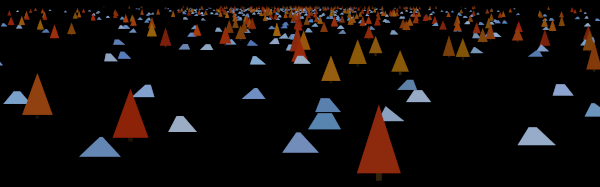if (R()<.2 && s+i>29)                  // is there an object?

{

// player object collision check

x = 2*roadWidth * R(10,-10) * R(9);  // choose object pos

const objectHeight = (R(2)|0) * 400; // choose tree or rock

if (!segment1.h                      // dont hit same object

&& Math.abs(position.x-x)<200                      // X

&& Math.abs(position.z-(s+i)*segmentLength)<200    // Z

&& position.y-height<segment1.y+objectHeight+200)  // Y

{

// slow player and mark object as hit

velocity = velocity.Multiply(segment1.h = collisionSlow);

}

const alpha = Lerp(i/drawDistance, 4, 0);  // fade in object

if (objectHeight)

{

// tree trunk

DrawPoly(x = p1.x+p1.z * x, p1.y, p1.z*29,

x, p1.y-99*p1.z, p1.z*29,

LSHA(5+R(9), 50+R(9), 29+R(9), alpha));

// tree leaves

DrawPoly(x, p1.y-R(50,99)*p1.z, p1.z*R(199,250),

x, p1.y-R(600,800)*p1.z, 0,

LSHA(25+R(9), 80+R(9), 9+R(29), alpha));

}

else

{

// rock

DrawPoly(x = p1.x+p1.z*x, p1.y, p1.z*R(200,250),

x+p1.z*(R(99,-99)), p1.y-R(200,250)*p1.z, p1.z*R(99),

LSHA(50+R(19), 25+R(19), 209+R(9), alpha));

}

}

}

}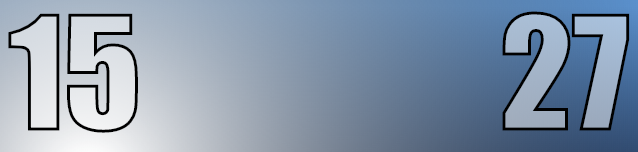if (mousePressed)

{

time = Clamp(time - timeDelta, 0, maxTime); // update time

DrawText(Math.ceil(time), 9);               // show time

context.textAlign = 'right';                // right alignment

DrawText(0|position.z/1e3, c.width-9);      // show distance

}

else

{

context.textAlign = 'center';      // center alignment

DrawText('HUE JUMPER', c.width/2); // draw title text

}

requestAnimationFrame(Update); // kick off next frame

} // end of update function

HTML 需要一个结束脚本标签来让所有的代码能够跑起来。

Update(); // kick off update loop

</script>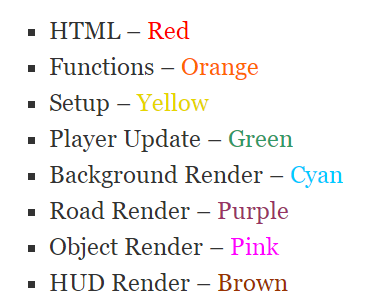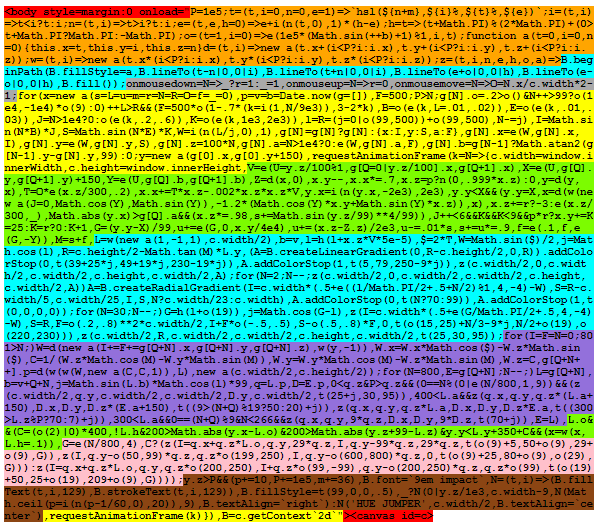Frank Force 在个人博客发了这篇文章后，在内容、标题的加持下，这篇文章后来被不少国外媒体转载。在盛赞之余，也有质疑的声音。网友“Anon”在原文下评论：你是如何在 2KB 安装一个完整的 javascript 的，除非你可以随意忽略 dependencies 插件库的大小，或者你将整个游戏作为 dependency，大小才有可能控制到 2KB，否则就是欺骗。

Frank 回复表示，大多数 small demos 都需要某种运行环境，即使它是可执行的。在这种情况下，就是 javascript 运行时环境，没有其他 dependencies.。因为 javascript 是解释的，所以也可以说压缩后的代码是在2KB以内的。

2 (3%)

加载中...

## 今日最热

加载中...

### 新品速递招聘